# Algebraic Distance

Bob Fisher

A linear distance metric commonly used in computer vision applications because of its simple form and standard matrix based least mean square estimation operations.

If a curve or surface is defined implicitly by(e.g.for a hyperplane) the algebraic distance of a pointto the surface is simply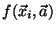.

If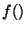can be factored as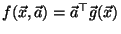(e.g.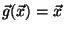for a hyperplane and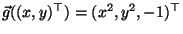for a circle) then the least square estimation of the parameters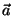is as follows. (This factoring is straightforward if the components of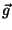are polynomials in the components of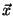.) Let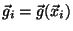. Then we wish to estimate thethat minimizesForm the matrix. Then the above equation can be reformulated as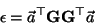The eigenvector corresponding to the smallest eigenvalue of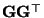is the parameter vectorthat minimizes the algebraic distance.

The algebraic distance is the same as the Euclidean distance for hyperplanes, but can have significant error for curved surfaces. As a consequence, Taubin produced a variant to the algebraic distance that may give parameter estimates with lower Euclidean distance. This approach is a first order approximation to the Euclidean distance and minimizes:is found using a generalized eigenvalue method. For more details on this distance and how to minimize it in closed form, see:

G. Taubin, F. Cukierman, S. Sullivan, J. Ponce, D.J. Kriegman. Parameterized families of polynomials for bounded algebraic curve and surface fitting. IEEE. Trans. Pat. Anal. and Mach. Intel., March 1994 (Vol. 16, No. 3) p.287-303.

Bob Fisher 2005-01-19